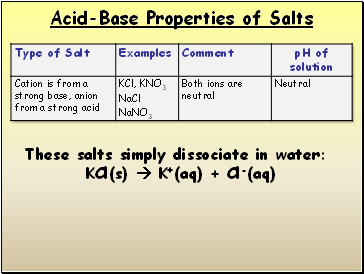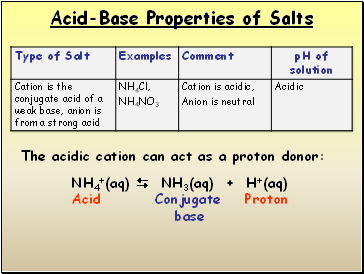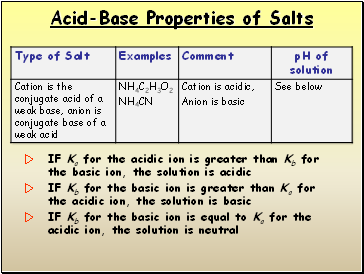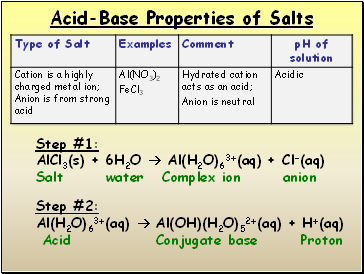# Acid-Base Properties of SaltsPage 1

#### WATCH ALL SLIDES

Slide 1## Acid-Base Properties of Salts

Slide 2Acid-Base Properties of Salts

These salts simply dissociate in water:

KCl(s)  K+(aq) + Cl-(aq)

Slide 3Acid-Base Properties of Salts

C2H3O2- + H2O  HC2H3O2 + OH- base acid acid base

The basic anion can accept a proton from water:

Slide 4Acid-Base Properties of Salts

NH4+(aq)  NH3(aq) + H+(aq)

Acid Conjugate Proton

base

The acidic cation can act as a proton donor:

Slide 5Acid-Base Properties of Salts

IF Ka for the acidic ion is greater than Kb for the basic ion, the solution is acidic

IF Kb for the basic ion is greater than Ka for the acidic ion, the solution is basic

IF Kb for the basic ion is equal to Ka for the acidic ion, the solution is neutral

Slide 6Acid-Base Properties of Salts

Step #1:

AlCl3(s) + 6H2O  Al(H2O)63+(aq) + Cl-(aq)

Salt water Complex ion anion

Step #2:

Al(H2O)63+(aq)  Al(OH)(H2O)52+(aq) + H+(aq)

Acid Conjugate base Proton

Slide 7## Effect of Structure on Acid-Base Properties

Increasing Acidity

Hypochlorous

acid

Chlorous

acid

Chloric

acid

Perchloric

acid